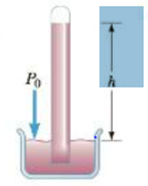Chapter 9, Problem 14P

Chapter
Section
Textbook Problem

Blaise Pascal duplicated Torricelli’s barometer using a red Bordeaux wine, of density 984 kg/m3 as the working liquid (Fig. P9.14). (a) What was the height h of the wine column for normal atmospheric, pressure? (b) Would you expert the vacuum above the column to be as good as for mercury?(a)

To determine
The height h of the wine column for normal atmospheric pressure.

Explanation
Assume that inside the tube the vacuum is created and outside of the tube means that surrounding area of the tube will have the non-zero atmospheric pressure and due to this pressure difference, the column would be filled with wine and the height of this wine column is defined as Patm=P+ρgh=0+ρgh .

Given info: Density of wine is 984kg/m3 , acceleration due to gravity is 9.80m/s2 , and the atmospheric pressure is 1.013×105Pa .

The formula the height of the column is,

h=Patmρg

• Patm is atmospheric pressure.
• ρ is density of the wine.
• g is acceleration due to gravity.

Substitute 984kg/m3 for ρ , 1

(b)

To determine
The vacuum above the column to be as good as for mercury.

Still sussing out bartleby?

Check out a sample textbook solution.

See a sample solution

The Solution to Your Study Problems

Bartleby provides explanations to thousands of textbook problems written by our experts, many with advanced degrees!

Get Started